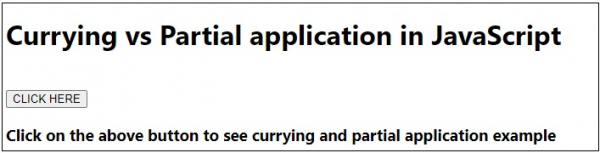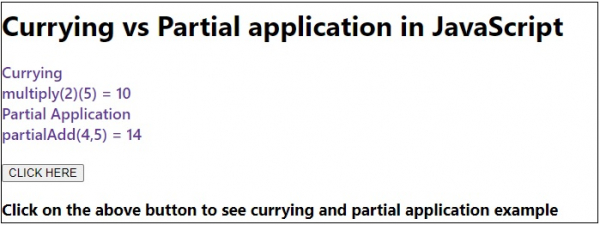# Currying VS Partial Application in JavaScript.

Currying − In currying a function takes another function and some arguments. The function then returns one function with one parameter only. It returns the function with one argument which can be chained together.

Partial application − In partial application some of the arguments can be bind to some values to produce a function with less arguments.

Following is the code for currying vs partial application in JavaScript −

## Example

Live Demo

<!DOCTYPE html>
<html lang="en">
<meta charset="UTF-8" />
<meta name="viewport" content="width=device-width, initial-scale=1.0" />
<title>Document</title>
<style>
body {
font-family: "Segoe UI", Tahoma, Geneva, Verdana, sans-serif;
}
.result {
font-size: 18px;
font-weight: 500;
color: rebeccapurple;
}
</style>
<body>
<h1>Currying vs Partial application in JavaScript</h1>
<div class="result"></div>
<br />
<h3>Click on the above button to see currying and partial application example</h3>
<script>
let resEle = document.querySelector(".result");
let BtnEle = document.querySelector(".Btn");
function multiply(x, y) {
return function (y) {
return x * y;
};
}
function add(a, b, c, d) {
return a + b + c + d;
}
resEle.innerHTML =
"Currying<br>multiply(2)(5) = " + multiply(2)(5) + "<br>";
resEle.innerHTML +=
"Partial Application <br> partialAdd(4,5) = " +
"<br>";
});
</script>
</body>
</html>

## Output